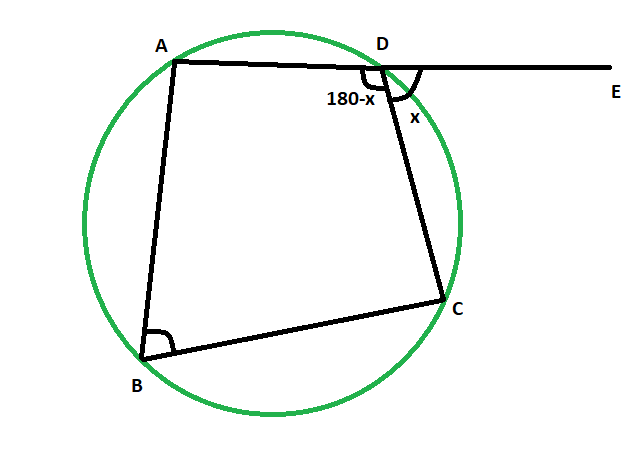Open in App
Not now

# Exterior angle of a cyclic quadrilateral when the opposite interior angle is given

• Last Updated : 30 May, 2022

Given cyclic quadrilateral inside a circle, the task is to find the exterior angle of the cyclic quadrilateral when the opposite interior angle is given.
Examples:

```Input: 48
Output: 48 degrees

Input: 83
Output: 83 degrees```Approach

• Let, the exterior angle, angle CDE = x
• and, it’s opposite interior angle is angle ABC
• as, ADE is a straight line
• so, angle ADC = (180-x) degrees
• since, opposite angles of a cyclic quadrilateral are supplementary,
• angle ABC = xBelow is the implementation of the above approach:

## C++

 `// C++ program to find the exterior angle` `// of a cyclic quadrilateral when` `// the opposite interior angle is given`   `#include ` `using` `namespace` `std;`   `void` `angleextcycquad(``int` `z)` `{` `    ``cout << ``"The exterior angle of the"` `         ``<< ``" cyclic quadrilateral is "` `         ``<< z << ``" degrees"` `<< endl;` `}`   `// Driver code` `int` `main()` `{` `    ``int` `z = 48;` `    ``angleextcycquad(z);` `    ``return` `0;` `}`

## Java

 `// Java program to find the exterior angle` `// of a cyclic quadrilateral when` `// the opposite interior angle is given`   `import` `java.io.*;`   `class` `GFG ` `{`   `static` `void` `angleextcycquad(``int` `z)` `{` `    ``System.out.print( ``"The exterior angle of the"` `        ``+ ``" cyclic quadrilateral is "` `        ``+ z +``" degrees"``);` `}`   `// Driver code` `public` `static` `void` `main (String[] args) ` `{` `        ``int` `z = ``48``;` `    ``angleextcycquad(z);` `}` `}`   `// This code is contributed by anuj_67..`

## Python3

 `# Python program to find the exterior angle` `# of a cyclic quadrilateral when` `# the opposite interior angle is given`   `def` `angleextcycquad(z):` `    ``print``(``"The exterior angle of the"``,end``=``""); ` `    ``print``(``"cyclic quadrilateral is "``,end``=``""); ` `    ``print``(z,``" degrees"``);`   `# Driver code` `z ``=` `48``;` `angleextcycquad(z);`   `# This code is contributed by 29AjayKumar`

## C#

 `// C# program to find the exterior angle` `// of a cyclic quadrilateral when` `// the opposite interior angle is given` `using` `System;`   `class` `GFG ` `{`   `static` `void` `angleextcycquad(``int` `z)` `{` `    ``Console.WriteLine( ``"The exterior angle of the"` `        ``+ ``" cyclic quadrilateral is "` `        ``+ z +``" degrees"``);` `}`   `// Driver code` `public` `static` `void` `Main () ` `{` `        ``int` `z = 48;` `    ``angleextcycquad(z);` `}` `}`   `// This code is contributed by anuj_67..`

## Javascript

 ``

Output:

`The exterior angle of the cyclic quadrilateral is 48 degrees`

Time Complexity: O(1)

Auxiliary Space: O(1)

My Personal Notes arrow_drop_up
Related Articles